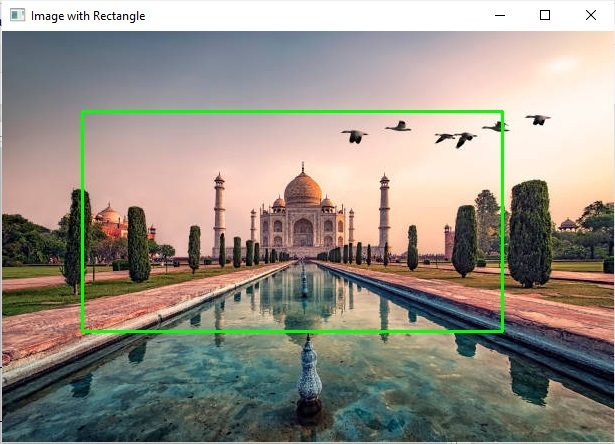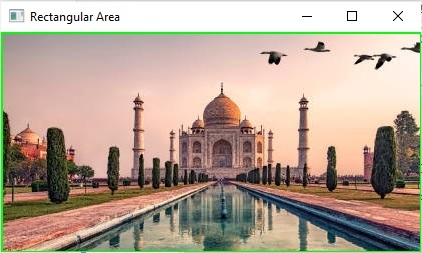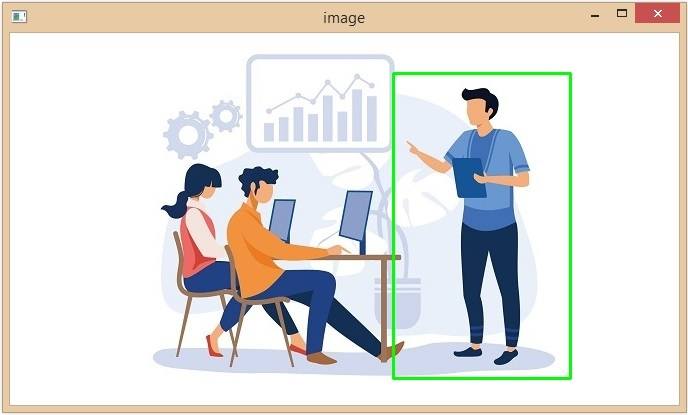# Draw a rectangular shape and extract objects using Python's OpenCV

OpenCV is an Open Source Computer Vision Library in python. It provides numerous functions to perform various Image and video processing operations. The library uses the Numpy module to represent all the video frames and images as a ndarray type. It needs the numpy library, we need to make sure that the numpy module is also installed in our python interpreter.

In this article, we will see different ways to draw rectangular shapes and extract the object using python OpenCV.

## Drawing a Rectangle

To draw the rectangular shape on an image, Python OpenCV module provides a method called cv2.rectangle(). This method will draw the rectangular shapes on an image. Following is the syntax –

cv.rectangle(img, pt1, pt2, color[, thickness[, lineType[, shift]]]	)


### Parameters

• img: The source image where to draw the rectangle.

• pt1: A tuple with the x and y coordinates of one of the vertices of the rectangle (the top left corner of the rectangle)

• pt2: A tuple with the x and y coordinates of the opposite vertex of the rectangle, regarding the previous one (the bottom right corner of the rectangle).

• color: It specifies the color of the rectangle.

• thickness: It is an optional parameter. It specifies the lines thickness of the rectangle. And the default thickness is 1.

x1,y1----------|
|		   |
| 		   |
| ------------x2,y2


Therefore the coordinates of pt1 pt2 will be (x1,y1) and (x2,y2) respectively.

## Using pre-defined dimensions

In this approach we will draw the rectangular shape on an image using the predefined co-ordinates. Which means we will define the pt1, pt2 value manually.

### Example

In this example, we will use the image co-ordinates to draw and extract the object from a rectangular shape.

import cv2
import numpy as np

# Define the dimensions and position of the rectangle using two points
top_left = (80, 80)
bottom_right = (500, 300)

# defining the colour and thickness of the rectangle
thickness = 2
color = (0, 255, 0) # Green color
shape = cv2.rectangle(img, top_left, bottom_right, color, thickness)

# Extracting objects from the rectangular area
rect_area = img[top_left:bottom_right, top_left:bottom_right]

# Display the image with the drawn rectangle
cv2.imshow("Image with Rectangle", img)

# Display the extracted rectangular area
cv2.imshow("Rectangular Area", rect_area)

cv2.waitKey(0)
cv2.destroyAllWindows()


### Output### Cropped Image## Using mouse event flags

To draw the rectangular shapes on an image, here we will use the below mouse event −

• cv2.EVENT_RBUTTONDOWN: indicates the right button is pressed.

• cv2.EVENT_LBUTTONUP: indicates the left button is released

Also, we will use the setMouseCallback() function to set the mouse event handler for the specified window

### The setMouseCallback() function

The function is used to specify which function has to be called for a specific window. In other words, the function creates a mouse event handler for the specified window.

### Syntax

cv2.setMouseCallback(winname, onMouse, userdata)


### Parameters

• Winname: Name of the specific window.

• OnMouse: Callback function for mouse events.

• Userdata: The optional parameter passed to the callback.

This approach can be executed using the command line interface. So here we will use the argparse module as it provides a convenient interface to handle the command line arguments.

Initially, we will set a mouse callback function to a namedWindow() method to read the rectangular coordinates where the user draws. And by using the mouse click events we will identify the x and y coordinates and then will draw the rectangular shape using the cv2.rectangle() function.

Note − To execute this code, we need to save the program file and input the image in the same location and then run the below command in the command prompt.

Python program_file_name.py --image source_image_name.jpg


### Example

Let’s take an example to draw the rectangular shape to extract the object.

import cv2
import argparse

point = []
crop = False

def shape_selection(event, x, y, flags, param):
# grab references to the global variables
global point, crop

# Record the starting(x, y) coordinates when the left mouse button was clicked
if event == cv2.EVENT_LBUTTONDOWN:
point = [(x, y)]

# check to see if the left mouse button was released
elif event == cv2.EVENT_LBUTTONUP:
# record the ending (x, y) coordinates
point.append((x, y))

# draw a rectangle
cv2.rectangle(image, point, point, (0, 255, 0), 2)
cv2.imshow("image", image)

# construct the argument parser and parse the arguments
ap = argparse.ArgumentParser()
ap.add_argument("-i", "--image", required = True, help ="Images/Dog.jpg")
args = vars(ap.parse_args())

clone = image.copy()
cv2.namedWindow("image")
# setting the mouse callback function
cv2.setMouseCallback("image", shape_selection)

# keep looping until the 'q' key is pressed
while True:
# display the image and wait for a keypress
cv2.imshow("image", image)
key = cv2.waitKey(1) & 0xFF

# press 'r' to reset window
if key == ord("r"):
image = clone.copy()

# if the 'c' key is pressed, break from the loop
elif key == ord("c"):
break

if len(point) == 2:
crop_img = clone[point:point, point:point]
cv2.imshow("crop_img", crop_img)
cv2.waitKey(0)

# close all open windows
cv2.destroyAllWindows()


Open the command prompt and execute the above program using the following command −

python test.py --image image5.jpg


This will generate a window displaying the input image on which you can select the desired object as shown below –Note − After selecting the desired area of the image press the key C on your keyboard to crop.We have successfully drawn the rectangular shape and extracted that selected object from the image.

Updated on: 30-May-2023

339 Views